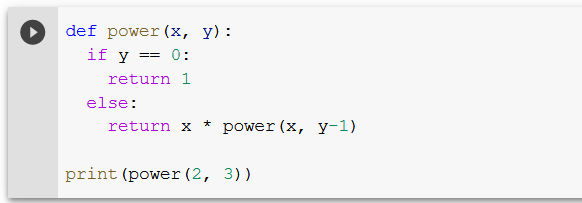# Recursive Power Function: Are You Stuck With This Sololearn Code?

Rate this post

If you learn Python with the excellent Sololearn app, you may find yourself with this code snippet:

```def power(x, y):
if y == 0:
return 1
else:
return x * power(x, y-1)

print(power(2, 3))```

What’s the output of this code snippet? And, most importantly, how does it work? This short guide will tell you!

The code creates a function that returns x^y. It leverages the important programming concept of recursion: it calls itself on a simpler version of the problem until it reaches a trivial case: `y=0`. We call this the recursion base case.

Base case: If the exponent `y` is 0, the solution is 1 because every number `x` taken to the power 0 is 1 by definition (see next section).

Non-base case: If the exponent `y` is larger than 0, the function `power(x, y)` returns the result of `x * power(x, y-1)`. It calls itself on a slightly easier problem. Say, you know the result of `power(x, y-1)`—that is `x^(y-1)`. You can now obtain `x^y` by multiplying the easier result (`x` to the power of `y-1`) with `x`. Here’s how that looks: `x^(y-1)*x = x^y`. You can see this idea in the following graphic:

Now, you can see how the code unfolds:Click to Run the Code in an Interactive Shell
• Variables x and y are defined. They’re `x=2` and `y=3`.
• Check whether `y = 0`, which is `False`, so you enter into the else branch.
• In the else branch, you call the function itself and repeat this check and computation to obtain the following steps:
```Step 0: x * (x^(y-1))
Step 1: 2 * (2^(3-1))
Step 2: 2 * (2^2)
Step 3: 2 * 4
Result: 8```

Thus, the output of this code snippet is 8.

## Why Does Any Number To The Power of Zero Gives One?

If you multiply any number with one, the number remains the same. For example, 1*x = x for all x. This property is called the multiplicative identity. Any number to the zero power is one because it’s just the product of no numbers at all, which is the multiplicative identity of 1. Similarly, the sum identity number is 0, so the sum of no numbers is the sum identity number 0.